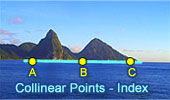# Collinear Points, Theorems and Problems - Table of Content 1Lemoine Line Theorem, Interactive illustration.
HTML5 Animation for iPad and Nexus

Nine-Point Center, Nine-Point Circle, Euler Line.
Interactive illustration.
Collinear Points.

Dynamic Geometry 1476.
Droz-Farny Line Theorem, Triangle, Orthocenter, Perpendicular, Collinear Midpoints, Step-by-step Illustration. GeoGebra, iPad.

Geometry Problem 1466.
Tangential Quadrilateral, Newton Line, Incenter, Midpoint, Diagonal, Collinear Points. Step-by-step Illustration using GeoGebra.

Geometry Problem 1462.
Newton-Line, Newton-Gauss Line, Complete Quadrilateral, Midpoints of Sides and Diagonals, Collinear Points.

Dynamic Geometry 1460.
Newton-Gauss Line, Complete Quadrilateral, Midpoints of Diagonals, Collinear Points, Step-by-step Illustration using GeoGebra.

Geometry Problem 1454.
Intersecting Circles, Perpendicular Lines, Cyclic Quadrilateral, Collinear Points, iPad. Step-by-step illustration using GeoGebra.

Dynamic Geometry 1450.
Ortholine, Steiner Line, Complete Quadrilateral, Collineal Orthocenters. Step-by-step illustration using GeoGebra.

Dynamic Geometry 1449.
Salmon Line. Step-by-step illustration using GeoGebra.

Pappus Theorem. Dynamic Geometry.
Step-by-Step construction, Manipulation, and animation.
Collinear Points.

Interactive Gergonne Line and Nobbs Points. Dynamic Geometry.
Step-by-Step construction, Manipulation, and animation.
Collinear Points.

Dynamic Geometry 1448.
Simson Line. Step-by-step illustration using GeoGebra.

Interactive Simson Line. Dynamic Geometry.
Step-by-Step construction, Manipulation, and animation.
Collinear Points.

GeoGebra, Dynamic Geometry: Lemoine Line of a Triangle.
HTML5 Animation for Tablets (iPad, Nexus..)

GeoGebra, Dynamic Geometry: Simson Line of a Triangle.
HTML5 Animation for Tablets (iPad, Nexus..)

Sawayama -Thebault's theorem
Collinear Points.

Monge & d'Alembert Three Circles Theorem II with Dynamic Geometry. You can alter the geometric construction dynamically in order to test and prove (or disproved) conjectures and gain mathematical insight that is less readily available with static drawings by hand.
Collinear Points.

Monge & d'Alembert Three Circles Theorem I with Dynamic Geometry. You can alter the geometric construction dynamically in order to test and prove (or disproved) conjectures and gain mathematical insight that is less readily available with static drawings by hand.
Collinear Points.

Triangle with the bisectors of the exterior angles. Collinearity.
Key concept: Menelaus Theorem.
Collinear Points

Step-by-Step construction, Manipulation, and animation
Collinear Points.

Triangle and Squares Theorem 1: with Interactive Geometry Software
Step-by-Step construction, Manipulation, and animation.
Collinear Points.

Newton's Theorem, Newton-Gauss Line: Complete quadrilateral theorem. Using TracenPoche Dynamic Geometry Software, Online Step-by-Step construction, manipulation, and animation.
Collinear Points.

Schiffler Point: Four Euler Lines with interactive animation and manipulation.
Collinear Points.

Newton's Theorem: Newton's Line. Circumscribed quadrilateral, midpoints of diagonals, center of the circle inscribed.
Collinear Points.